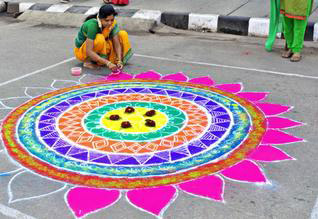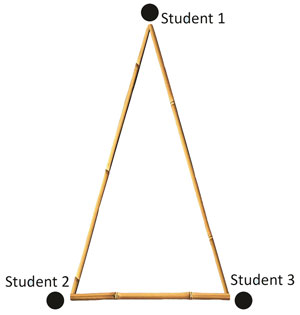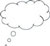# 3 Developing geometric intuition

Intuitive understanding of geometry develops from experiences in real life, and often involves dynamic images. The shapes we use and play with are not static: they change, they move and they can be transformed into other shapes. For example, when sharing a square piece of cake with a friend (equally of course!), the square can be transformed into two triangles, two rectangles, etc. When making rangoli patterns (see Figure 3), shapes within shapes are played with, changed and explored.Figure 3 A rangoli being drawn.

Similar playfulness with shapes can be found in architecture. In contrast, geometry in school mathematics is often perceived as something static: facts that are not possible to play with and change. In recent years that perception is slowly being addressed by the development of dynamic geometry software such as Cabri or the free shareware package GeoGebra.

So in addition to exploring ways to overcome the barrier of the language used in school geometry, you are now going to think about developing geometric intuition. Fujita et al. (2004) describe geometric intuition as involving:

the skills to create and manipulate geometrical figures in the mind, to see geometrical properties, to relate images to concepts and theorems in geometry, and decide where and how to start when solving problems in geometry.

Like Part 2 of Activity 1, this will focus on variance and invariance; that is, on what stays the same and what can change. The next activity gives an example of how students could work more intuitively on geometrical statements and develop dynamic geometrical images in the mind at the same time. They will do this by thinking about the statement: ‘Equal chords of a circle subtend equal angles at the centre.’

## Activity 2: An intuitive approach to thinking about geometrical statements

For this activity, students need three straight long sticks such as bamboo poles, which are not the same length. Ask them to use these sticks to create a triangular frame so that two of the sides of the frame are equal – in other words, to create an isosceles triangle (see Figure 4). This activity is best done outside, if possible, where the students can trace their movements in some soil or sand.Figure 4 An isosceles triangle.

### Part 1: Seeing what is happening

• Ask each of the students holding the bamboo poles to say something about the sticks (or sides of the triangle) that they are holding. Can they say something about the angle or relative length? What is the same as and what is different from the other sides or angles?
• Now ask the students to move the sides of the triangle. Tell Student 1 to stay in the same position, although they can turn. Ask Students 2 and 3 to move the triangle, leaving Student 1 in the same place.
• Ask the other students the following questions:
• What shape is formed when tracing the movements of Student 2, then Student 3? (Answer: a circle – and both students are forming the same circle.)
• Why is this shape formed?
• What role is Student 1 enacting? (Answer: the centre of the circle.)
• How they could describe the distance between Students 2 and 3? (Answer: a chord of the circle.)
• How they could describe the distance between Students 1 and 2 or Students 1 and 3? (Answer: the radius.)

### Part 2: Working on invariance

Ask the students first to discuss in threes and be ready to share their thinking about these three questions with the rest of the class:

• In what ways could the length of the bamboo sticks be changed?
• What else would change in these cases? What would stay the same?
• Can they make a mathematical statement that would describe what they think?

### Part 3: Making sense of the mathematical statement ‘Equal chords of a circle subtend equal angles at the centre’

While still outside, give the students the statement ‘Equal chords of a circle subtend equal angles at the centre’ in some written format, because it is very difficult to remember.

• Ask the students to discuss in groups of three what this statement could mean. How does it relate to what they have been working on? Remind them they can try it out by using the bamboo sticks or in some other way.
• Ask the students for suggestions on how such mathematical statements could be made more accessible.
• Have a whole-class discussion about their thoughts.Video: Using local resources

You can read more in Resource 2, ‘Using local resources’.

## Case Study 2: Mrs Chakrakodi reflects on using Activity 2

For this activity we went out in the field. First we did Part 1 together as a whole class. Because I had enough bamboo sticks for five groups, I divided the students into five groups and gave them three pieces of bamboo, two of which were of the same length, and one that was different for each group. They were then told to do Part 1 of the activity within their groups and mark out the path it made. I also asked them to make notes of their observations.

The third stick of each group was then exchanged several times (they were all of different lengths) and they did the same activity again. I decided to do this before asking the questions from Part 2 of the activity, because I thought this might lead them to actually come up with similar questions. Then by the time I asked them the questions of Part 2, they would feel good, as it would be questions they had thought of already – which indeed was the case to some extent.

For Part 2 I asked them to work in groups of three at first because I thought working in smaller groups would help the mathematical discussion. They found making mathematical statements hard, but they had a go at it. It provided good preparation for Part 3 of the activity. To make sense of the vocabulary of the statement ‘Equal chords of a circle subtend equal angles at the centre’, it was really helpful to do that part of the activity in the same physical setting because it allowed the students to really point to what they had done earlier, try out some ideas and relate their vocabulary to that of the given statement.

There was a lot of running around and movement. After they had all noted down what they observed and had some whole-group discussions, we went back to the classroom. Once there, they sat down to have a further discussion of what had happened and what it meant. Although some part of that discussion repeated what was said earlier, I thought it important to make the link explicit between what happened outside the classroom and what they had to do sitting in their desks, surrounded by textbooks and exercise books. As part of their home activity I asked them to think of some activity for the other properties they had already discussed in Activity 1.Pause for thought What responses from students were unexpected? Why?What questions did you use to probe your students’ understanding? Did you feel you had to intervene at any point?

2 The circle in life versus the circle in mathematics: vocabulary

4 Making up own questions to develop geometric imagery